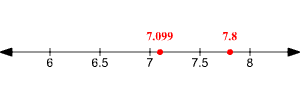# Comparing Decimals

To compare two decimal numbers, first see if the integer parts are different. If they are, then the number with the greater integer part is greater.

If the integer parts are the same, then check if they have the same number of digits to the right of the decimal point. If they don't, write additional zeros on the right side of one of the numbers until they do.

Example 1:

To compare $7.8$ with $7.099$ , first write $7.8$ as $7.800$ . Now the two numbers both have $3$ digits to the right of the decimal point.

Next, starting from the left, compare the digits until you find the larger number.

Example 2:

Comparing $7.800$ with $7.099$ , we find the first digit that differs is the tenths place. The digits in this position are $8$ and $0$ . Since $8$ is greater than $0$ ,

$7.8>7.099$ .

You can think of plotting the numbers on the number line. The number to the right is greater than the number to the left.Another way to think of this is to convert the decimals to fractions with the same denominator.

$\begin{array}{l}7.8=7.800=\frac{7800}{1000}\\ 7.099=\frac{7099}{1000}\end{array}$

Then just compare the numerators.